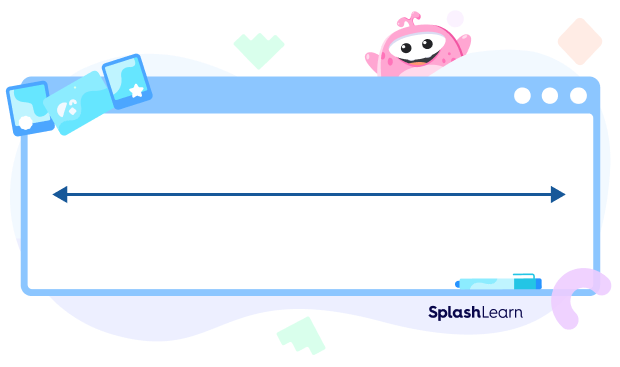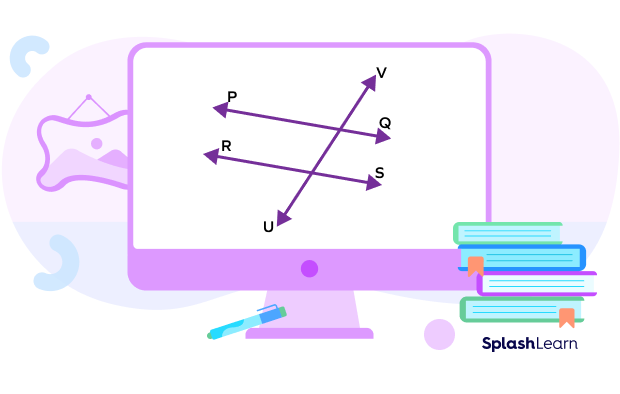# Types of Lines – Definition With Examples

## Definition of a Line

You can observe lines all around you: from the ruler in your pencil box to train tracks, from the buildings in the city to the walls in your home. Lines are a rich and fascinating concept in mathematics, and there is a lot to learn about them.

Mathematically, a line is a collection of infinite points. In addition, a line extends endlessly in both directions. It is a one-dimensional figure with no end, both to the left and to the right.

## Diagram of a Line

It does not have any endpoint. The two arrows at each end signify that the line extends endlessly and is unending in both directions. The length of a line cannot be measured.

All geometric shapes, such as angles, line segments, polygons, etc., are made up of lines. With the help of lines, points and angles, you can determine figures and shapes.

## What are the Types of Lines in Geometry?

There are various types of lines used in everyday life. Getting to know the various types of lines helps us to develop a sound understanding of the figures and shapes in math. Let’s look at the geometry line names and the examples  of types of lines in everyday life.

### a) Curved Lines:

A line that is not straight is a curved line. If a point does not move in one direction, we get a curve.

### b) Straight Lines:

The shortest line joining any 2 points is a straight line. If a point moves in only one direction, we get a straight line.

### c) Horizontal Lines:

A horizontal line is a line that runs from left to right in a straight line. Horizontal lines are parallel to the x-axis and perpendicular to the y-axis.

### d) Vertical Lines:

Vertical lines run straight up and down from top to bottom or bottom to up. Vertical lines are parallel to the y-axis and perpendicular to the x-axis.

### e) Parallel Lines:

In geometry, parallel lines can be defined as two lines in the same plane that are at equal distance from each other and never meet.

### f) Intersecting Lines:

When two or more lines cross each other in a plane, they are called intersecting lines. The intersecting lines share a common point, which lies on all the intersecting lines.

### g) Perpendicular Lines:

In geometry, perpendicular lines are defined as two lines that meet or intersect each other at a right angle (90°).

### h) Transversal Lines:

A line that intersects two or more given lines at different points is called a transversal line.

## Solved Examples

Example 1:  Match the figures with the types of lines.

Solution: The types of lines for the figures are as follows:

1. Parallel lines
2. Intersecting lines
3. Curved line
4. Vertical line

Example 2: State whether the following is true or false.

1. A line is a set of points in a straight path that extends in opposite directions without ending.
2. A line has a fixed length.
3. Horizontal lines are parallel to y-axis.
4. A ray extends in one direction only.

Solution:

1. True
2. False
3. False
4. True

Example 3: Write the name of a given line, ray, or line segment.

Solution:

1. $XY^\leftrightarrow, YX^\leftrightarrow$
2. $\overline{UV}$
3. $\overrightarrow{AB}$

Example 4: Observe the given figure and answer the given questions.

1. Name the ray.
2. Name the line.
3. Name the line segment.

Solution:

1. $\overrightarrow{DC}$
2. $AB^\leftrightarrow$
3. $\overline{EF}$

## Practice Problems

1

### A ray can be defined as a line with ___________.

no endpoints
two endpoints
four endpoints
one endpoint
CorrectIncorrect
A ray can be defined as a line with one endpoint.
2

### What is the direction of horizontal lines?

Slanted
Curve
Top to bottom
Left to right
CorrectIncorrect
Correct answer is: Left to right
A horizontal line goes from left to right.
3

### Write the name of the figure.Line
Roy
Line segment
Curved line
CorrectIncorrect
A line extends in both directions infinitely.
4

### Name the transversal line in the given figure.PQ
RS
UV
None of the above
CorrectIncorrect
UV intersects RS and PQ at different points. So, UV is a transversal line.